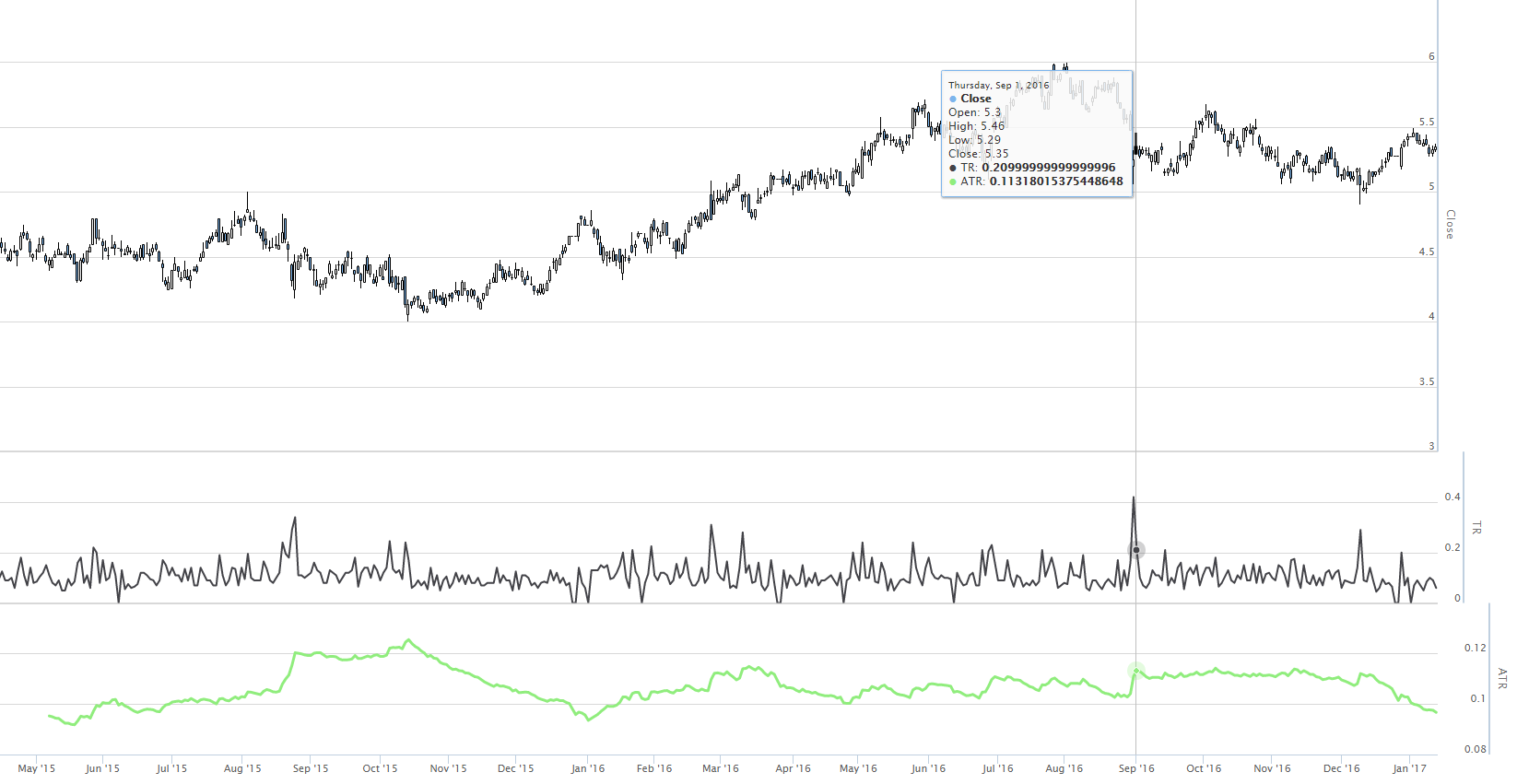Average true range is an indicator that is useful for judging the volatility of a stock. I use this to set the level for my stop loss orders. In this post I'm going to show how to calculate and visualise average true range in JavaScript.

# Getting the code

Working example code for this post is available to Patrons.

# Getting the code running

After cloning or downloading and unpacking the code, change to the repo's directory and install dependencies via npm:

``cd average-true-range-demonpm install``

Then change into the the `client` directory and install bower dependencies:

``cd clientbower install``

To start the server change back to the previous directory and run it using Nodejs:

``cd ..node index``

You can now browse to http://localhost:3000 and view the web page.

# What is average true range?

Average true range is an indicator that measures the trading range of a stock and shows how volatile it is.

# Screenshot

This is what the end result of this post looks like:# Computing true range

Average true range first requires that we compute true range for a stock.

True range is the maximum of the values evaluated from the following formulas:

``````High - Low
High - Yesterday's Close
Yesterday's Close - Low
``````

The data we need for this is today's high and low and yesterday's close for the stock we are interested in.

We can represent this in JavaScript as follows:

``var high = ...var low = ...var yesterdaysClose = ...var trueRange = Math.max(    high - low,     high - yesterdaysClose,     yesterdaysClose - low);``

# Generating ATR with Data-Forge

To generate average true range for a stock we must calculate true range over the stock history. Then we can average this value over time.

In this example data is acquired from Yahoo and downloaded to a Data-Forge dataframe as demonstrated in my previous post.

The `rollingWindow` method is used to walk across the dataframe looking at two days at a time, yesterday and today, to compute a true range time series:

``var ohlc = ... dataframe downloaded from yahoo ...// Compute time series for true range...var trueRange = ohlc    .rollingWindow(2)    .asPairs()    .select(function (window) {        var today = window.last();        var yesterday = window.first();        var trueRange = Math.max(            today.High - today.Low,            today.High - yesterday.Close,            yesterday.Close - today.Low        );        return [            window.last(),            trueRange,        ];    })    .asValues();    ``

`asPairs` is used to extract the sequence as index/value pairs, so that we can rewrite the index of the time series. `asValues` finally brings it back to a sequence of values.

Once we have the true range as a time series, we can use the `ema` function from data-forge-indicators to compute an exponential moving average:

``````var averageTrueRange = trueRange.ema(atrPeriod);
``````

It isn't difficult then to visualize this in graph using Highstock, I did something very similar in the previous post.

The time series is converted to Highstock format using data-forge-to-highstock as follows:

``var highstockData = averageTrueRange.toHighstock()``

# Resources

Data-Forge: https://www.npmjs.com/package/data-forge

Highstock Demos: http://www.highcharts.com/stock/demo